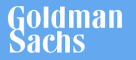New update is available. Click here to update.
Topics

# Count Triplets

Easy0/40
Average time to solve is 15m+19 more companies

## Problem statement

You have been given an integer ‘X’ and a non-decreasing sorted doubly linked list with distinct nodes.

Your task is to return the number of triplets in the list that sum up to the value ‘X’.

Detailed explanation ( Input/output format, Notes, Images )
Constraints :
``````1 <= T <= 5
1 <= N <= 10 ^ 3
- 3 * 10 ^ 5 <= X <= 3 * 10 ^ 5
- 10 ^ 5 <= data <= 10 ^ 5 and data != - 1

Where ‘N’ is the number of nodes in the given linked list, and ‘X’ is the required triplet sum value.

Time limit: 1 sec
``````
Sample Input 1:
``````2
13
-4 2 3 8 9 -1
5
-4 -2 3 4 5 -1
``````
Sample Output 1:
``````2
2
``````
Explanation to Sample Input 1:
``````In the first test case, there are two possible triplets {2,3,8} and {-4,8,9}, whose sum is 13.
In the second test case there are two triplets possible {-4,4,5} and {-2,3,4} whose sum is 5.
``````
Sample Input 2:
``````2
-4
-3 8 10 -1
12
1 4 6 2 -1
``````
Sample Output 2:
``````0
1
``````
Explanation to Sample Input 2:
``````In the first test case, there is no triplet whose sum is -4.
In the second test case, there is only one triplet {6,4,2}, whose sum is 12.
``````Console